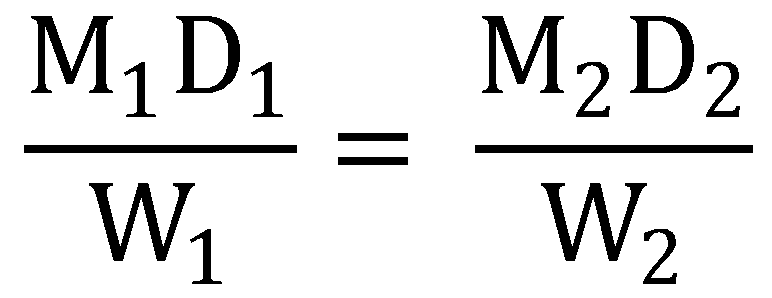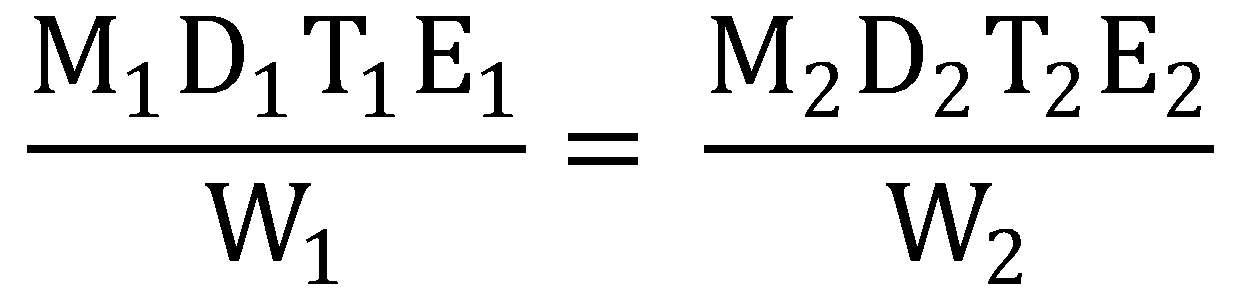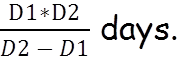# Time & Work

Rule 1: Universal Rule
This rule can be used in almost every problems.

If M1 persons can do W1 work in D1 days and M2 persons can do W2 works in D2days then we can sayM1D1W2 = M2D2W1

If the persons work T1 and T2 hours per day respectively then the equation gets modified toM1D1T1W2 = M2D2T2W1

If the persons has efficiency of E1 and E2 respectively then,M1D1T1E1W2 = M2D2T2E2W1

Rule 2:
If A can do a piece of work in n days, then
The work done by A in one day = 1/n

Rule 3:
If A can do a work in D1 days and B can do the same work in D2 days then A and B together can do the same workRule 4:
If A is twice as good a workman as B, then A will take half of the time taken by B to complete a piece of work.

Rule 5:
If A is thrice as good a workman as B, then A will take one third of the time taken by B to complete a piece of work.

Rule 6:
If A and B together can do a piece of work in x days, B and C together can do in y days and C and A together can do in z days, then the same work can be doneRule 7:
If A can do a piece of work in D1 days, B can do in D2 days and C can do in D3days then they together can do the same work inRule 8:

If A and B together can do a piece of work in D1 days and A alone can do it in D2days, then B alone can do the work inRule 9:
If the number of men are changed in the ratio of m:n, then the time taken to complete the work will change in the ratio n:m